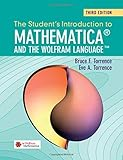# The Student’s Introduction to Mathematica and the Wolfram Language, 3rd EditionThe Student's Introduction to Mathematica and the Wolfram Language

By 作者: Bruce F. Torrence - Eve A. Torrence
ISBN-10 书号: 110840636X
ISBN-13 书号: 9781108406369
Edition 版本: 3

Release Finelybook 出版日期: 2019-06-27
Paperback :(544 )
<

Book Description to Finelybook sorting

The unique feature of this compact student’s introduction to Mathematica® and the Wolfram Language(TM) is that the order of the material closely follows a standard mathematics curriculum. As a result, it provides a brief introduction to those aspects of the Mathematica® software program most useful to students. Used as a supplementary text, it will help bridge the gap between Mathematica® and the mathematics in the course, and will serve as an excellent tutorial for former students. There have been significant changes to Mathematica® since the second edition, and all chapters have now been updated to account for new features in the software, including natural language queries and the vast stores of real-world data that are now integrated through the cloud. This third edition also includes many new exercises and a chapter on 3D printing that showcases the new computational geometry capabilities that will equip readers to print in 3D.

The Student’s Introduction to Mathematica and the Wolfram Language, 3rd Edition 9781108406369.pdf

### 觉得文章有用就打赏一下文章作者

#### 支付宝扫一扫打赏#### 微信扫一扫打赏• QQ咨询
• 回顶